## Example Questions

### Example Question #102 : Arithmetic

The sequenceis defined as:What is the difference between the 20th and the 30th elements of?The answer cannot be determinedExplanation:

For this sequence, you do not have a starting point (i.e.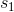); however, you are able to interpret it relatively easily. The sequence is merely one in which each number is twenty larger than the one preceding it. Therefore, ifwere, you would have: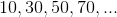Now, to find difference between the 20th and the 30th element, it is merely necessary to count the number of twenties that would be added for each of those elements. For instance, the difference between the 21st and the 20th elements is. Thus, since you would add a total of ten twenties from the 20th to the 30th element, you know that the difference between these two values is.

### Example Question #2 : How To Find The Common Difference In Sequences

Which of the following defines any term in a linear sequence havingfor its first term and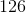for its ninth term?Explanation:

Since this sequence is linear, we know that it will add the same amount for each element. This means that you can evenly divide the difference between the first and the ninth term. Be careful! There will be eight total increases between these terms. (Think this through: 1 to 2, 2 to 3, 3 to 4, etc.)

Thus, we know that the total difference between these terms is: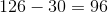Now, dividing this among the eight increases that happen, we know: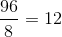This means that for each element, we addto the one prior to it. This means that our general sequence is defined as: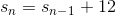### All GRE Math Resources